Function Repository Resource:

# MissingDataLogLikelihood

Compute a log-likelihood for data with missing values

Contributed by: Sjoerd Smit
 ResourceFunction["MissingDataLogLikelihood"][dist, data] computes the log-likelihood for obervations data from the distribution dist, assuming that the probabilities that values are Missing is independent of the observed values.

## Details

ResourceFunction["MissingDataLogLikelihood"] uses MarginalDistribution to obtain distributions for data rows where elements are Missing.

## Examples

### Basic Examples (2)

Compute a log-likelihood from data with missing values:

 In:=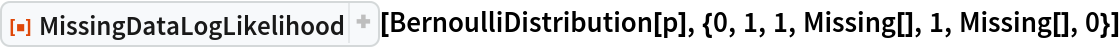Out=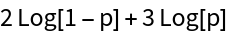Since this is univariate data, this is equivalent to:

 In:=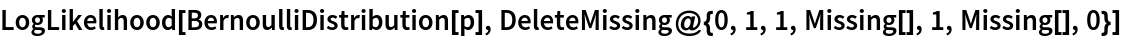Out=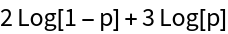Compute a log-likelihood for a multivariate distribution:

 In:=Out=### Applications (1)

Find a maximum-likelihood estimate for a BinormalDistribution with randomly missing values:

 In:=In:=Out=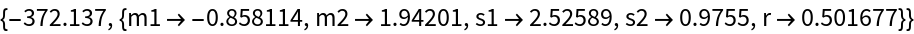Sjoerd Smit

## Version History

• 1.0.1 – 21 June 2023
• 1.0.0 – 07 June 2023